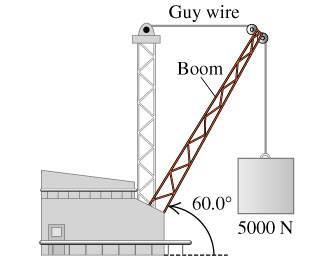# Statics:Find the Tension in a rope holding a boom

• fishturtle1

## Homework Statement

The boom in the figure below (Figure 1) weighs 2450 N and is attached to a frictionless pivot at its lower end. It is not uniform; the distance of its center of gravity from the pivot is 35.5 % of its length.
Find the tension of the guy wire.Στ=0
∑F=0
τ=F*Dsin(θ)

## The Attempt at a Solution

I made a free body diagram where the boom is the object and i drew three forces acting on it.

The three forces acting on the boom are weight of the boom, weight of the box, and the tension in the rope.

I then tried to use the sum of the torques to find tension:

Στ=0=wboomsin(60°)(.355L) + wboxsin(60°)L - T1sin(30°)L

then rearranged

wboomsin(60°)(.335L) + wboxsin(60°)L = T1sin(30°)L

then i canceled out the L variable, and plugged in and simplified and got this:

T1 = 10,167 N => 10200 N.

This is wrong.

I peaked at the yahoo answer and they used cos(θ) instead of sin(θ) which does not make sense to make because then the force calculated would move the boom linearly rather than creating a torque.

Last edited:

## Homework Statement

The boom in the figure below (Figure 1) weighs 2450 N and is attached to a frictionless pivot at its lower end. It is not uniform; the distance of its center of gravity from the pivot is 35.5 % of its length.Στ=0
∑F=0
τ=F*Dsin(θ)

## The Attempt at a Solution

I made a free body diagram where the boom is the object and i drew three forces acting on it.

The three forces acting on the boom are weight of the boom, weight of the box, and the tension in the rope.

I then tried to use the sum of the torques to find tension:

Στ=0=wboomsin(60°)(.355L) + wboxsin(60°)L - T1sin(30°)L

then rearranged

wboomsin(60°)(.335L) + wboxsin(60°)L = T1sin(30°)L

then i canceled out the L variable, and plugged in and simplified and got this:

T1 = 10,167 N => 10200 N.

This is wrong.

I peaked at the yahoo answer and they used cos(θ) instead of sin(θ) which does not make sense to make because then the force calculated would move the boom linearly rather than creating a torque.
Post the entire problem statement.

I peaked at the yahoo answer and they used cos(θ) instead of sin(θ) which does not make sense to make because then the force calculated would move the boom linearly rather than creating a torque.
For the torque of a vertical force around the axis you need the horizontal distance from the axis to the line of action of the force.
If L is the hypotenuse and the angle to the horizontal is θ, what is that horizontal distance?

For the torque of a vertical force around the axis you need the horizontal distance from the axis to the line of action of the force.
If L is the hypotenuse and the angle to the horizontal is θ, what is that horizontal distance?
the horizontal distance would be Lcos(θ), but why are we treating L as a hypotenuse?

In my diagram I reoriented the boom horizontal and then i did sinθ to find the Fy of each force and then calculated the torques that way.

I am confused when you say "you need a horizontal distance from the axis of the line of action of the force"? So does this mean I need to find the distance from the axis of rotation to the line where the force is being applied?